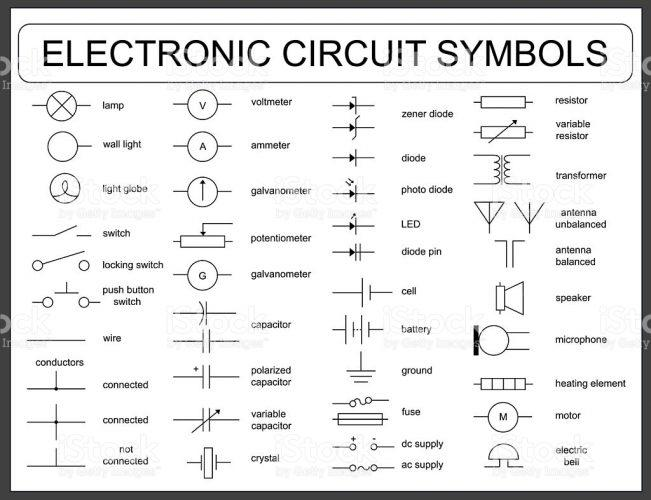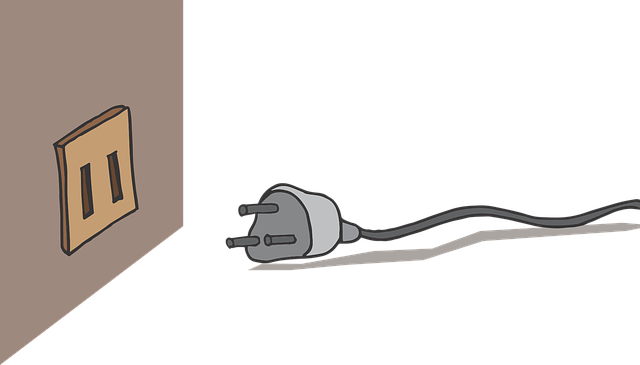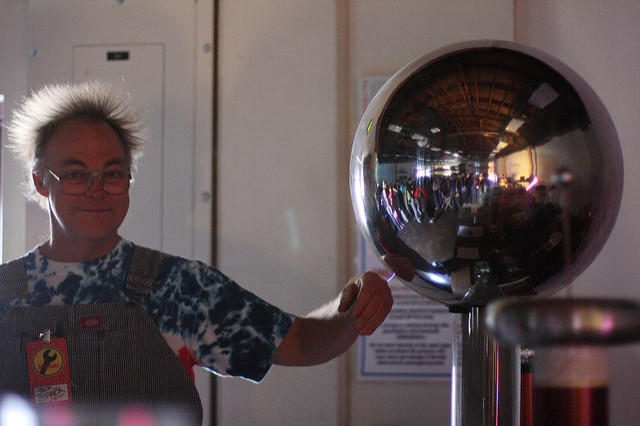"We forget just how painfully dim the world was before electricity. A candle, a good candle, provides barely a hundredth of the illumination of a single 100 watt light bulb." -Bill Bryson

Try to imagine a world without electricity: no light, no televisions, no heating during the winter or cooling in the summertime, no internet or no hot water while taking a shower.

In all honesty, the world would be a pretty grim place. Nevertheless, for centuries, men and women lived without this useful commodity. People in ancient times used candlelight and woodfires to accomplish daily tasks.

However, thanks to intelligent men who made scientific advancements and dedicated their lives to the study of various scientific disciplines the light began to shine brighter both figuratively and literally.

Through the study of Physics electricity can be thoroughly understood by students of all ages.

In England, Wales and Northern Ireland the GCSE Physics option is offered to students who are nearing graduation and who have scientific interests. The second topic of this Physics Syllabus is electricity and the basic concepts are taught to pupils to further their knowledge on the subject. Superprof is here to analyze the four sections covered in this second topic of the GCSE Physics subject.

## Electric Circuits

The AQA, a UK-based exam board, offers students a lot of instruction about Electricity in various sections. The first section has to do with electric circuits.

### Electrical Circuit Symbols

There are various symbols used to identify the different components that can be found in an electric circuit. Here are the most commonly observed symbols on an electric circuit:There are many electronic circuit symbols that can be studied to better understand an electric diagram. (Source: iStock)

Some of the most common components on an electric circuit include the following:

• Switch: a very simple component used to turn a circuit on and off.
• Lamp: an electric current heats a bulb so that it can give out some light.
• Fixed Resistor: restricts or limits the flow of electrical current. The fixed resistor has a resistance that will never change.
• Variable Resistor: used for some dimmer switches and volume controls. Moving the position of the slider causes it to change.
• Thermistor: resistance depends on the temperature. When the temperature is low the resistance is elevated and when the temperature is high the resistance decreases. Used in fire alarms or thermostats in the house.
• Light-dependent resistor (LDR): resistance depends completely on light intensity. On lower light levels, the LDR has a higher resistance and as the light increases the resistance decreases. Used as a sensor in cameras or automatic lights.
• Semiconductor diode: allows current to flow in only one direction and it will never flow in the opposite direction.

Check for a physics maths tutor here.

### Electrical Charge and Current

There are two types of electric current: direct and alternating. The characteristics of a direct current are that the electrons constantly flow in the same direction around a circuit. Whereas in an alternating current, the direction and flow of electrons change constantly and reverses.

A charge is a property which experiences a force in an electric field and they are measured by coulombs (C).

Since electrons are very small, a charge is equivalent to 6,250,000,000,000,000,000 electrons.

That's a lot of zeroes! These are all important details that can be learnt in the electricity topic of GCSE Physics.

Currents are essential in electric circuits. When the current flows through the circuit electrical work is done and energy is transferred. A very simple equation can be used to calculate the amount of charge going through a point of the circuit:

charge= current x time or Q = I x t

The charge (Q) is measured in coulombs, current (I) is measured in amps and time (t) is measured in seconds.

### Potential Difference

Students also learn a lot more about potential difference which is the measure of how much energy is transferred between two points on a circuit. To measure the potential difference of an electric circuit a voltmeter needs to be placed in parallel with the component that is being measured.

The potential difference can be calculated by using a basic equation such as the following:or(Both images are courtesy of bbc.com/bitesize/guides)

The potential difference (V) is measured using volts, energy (E) is calculated by using joules and charge (Q) is measured in coulombs.

### Series Circuits

Series circuits are also analyzed which is the fact that electrical components are connected one after another in a single loop. The circuit rules include the fact that an electron will pass through every component on its way around the circuit. If a bulb is broken the current will not be able to go around the whole circuit.

Some of the most basic characteristics about series circuits are taught which include the facts that the current is the same throughout each component of the circuit, the total potential difference is shared between all of the components and the total resistance of the circuit is the sum of an individual resistor.

### Parallel Circuits

While learning about parallel circuits, students acquire useful knowledge on the subject such as the fact that the total current provided is divided between the components on different loops, potential difference is spread equally and is the same across each loop and the total resistance of the electric circuit can be reduced as the current can follow different paths.

This information is attained by studying diagrams and analyzing the various equations and formulas.

### Energy and Power in Electric Circuits

The wires heat up after electrons flow through them and collide with the ions which cause them to vibrate. This vibration increases the temperature of the wire. More information and practise questions can be found online using trusted websites. Finding supplemental information helps students stay ahead of the game while studying physics.

### Required Practical

During this section of the topic of electricity, pupils must complete three required practical exercises:

• Investigate the factors that affect resistance: in this practical exercise, pupils work towards the aim of this experiment which is to investigate how changing the length of the wire affects its overall resistance.
• Investigate current-voltage graphs: in this scientific experiment, scholars observe a series of diagrams and then complete the nine steps that are part of the method.
• Investigate resistor networks: in this practical exercise, students discover that there is more than one way to investigate resistor networks. They record potential difference and current in an accurate manner and use appropriate apparatuses and methods to measure potential difference.

Your maths and physics tutor can help you understand the laws governing resistance and how to calculate it!

## Mains ElectricityAfter studying the electricity topic in GCSE Physics, you will never look at household plugs in the same way! (Source: pixabay)

As we saw in the previous section, electricity can flow as a direct or alternating current. We all need electricity in our homes to heat or cool and power our appliances.

A direct current would appear as a straight line on a voltage-time graph and an alternating current would look like a curve that changes between positive and negative voltages.

Students learn that in the United Kingdom the mains electrical supply is generated at a frequency of 50 Hertz (Hz) and is sent to houses at 230 Volts (V).

In every household, there are plugs that connect the mains electricity supply to a commonly used device. The cable of the plug contains copper wires, which are great conductors, that are coated with plastic and known as a fantastic insulator.

Each plug has many parts and every part has its own function:

• Outer insulation: the three copper wires are safely wrapped with extra plastic insulation to avoid any serious accidents.
• Cable grip: holds the cable tightly into place so that the wires do not become loose.
• Livewire: a copper wire that is coated with brown plastic where the current enters the device.
• Fuse: made of glass or ceramic and contains a thin wire that melts if the current gets too hot. If the fuse is melted the circuit is broken and no more current flows through the device.
• Neutral wire: a copper wire covered with blue plastic that connects the cable in the wall and completes the entire circuit.
• Earth wire: a copper wire coated in plastic that provides a way for the current to flow from the case of the device to the ground if there is a fault. This is a very important aspect because it prevents a person from getting electrocuted the next time they touch the faulty appliance.

### Electrical Appliances

All appliances transfer energy from one store to another. The amount of energy transferred highly depends on the power and the amount of time. This can be calculated using the following equation:

energy= power x time or E = P x t

Energy (E) is measured in joules, power (P) is calculated in watts and time (t) is calculated in seconds.

### Alternating Current and the National Grid

The National Grid distributes electricity all over the country. The electricity is produced by a conventional power station by turning a generator or using another popular method.

Transformers are utilized to change voltages and currents in transformation lines. They are used by the National Grid to increase the voltage and reduce the current. When the current is lowered it means less energy is lost when heating the wire.

This is done to make sure that the grand majority of energy is transferred to the consumer's house rather than be wasted on the heating from the wires.

Master these concepts and more with your maths and physics tutor!

## Static ElectricityA Van de Graaf generator produces a positive charge and a person does not have to touch one in order to feel the effects. (Source: Visual Hunt)

The motion of charged particles, such as rubbing two things together, causes electrical effects like small shocks, lightning and sparks.

Atoms can be observed in all matter and atoms contain three types of smaller particles such as protons, neutrons and electrons.

Protons are positively charged and electrons are negatively charged. Certain objects that are charged can affect other charged objects using the non-contact forces of static electricity.

### Friction

Friction is caused when insulating materials are rubbed against each other. For example, when a polythene rod is rubbed with a duster, the friction causes electrons to gain energy. Insulators prevent the electrons from moving forward and therefore the charge becomes static.

The properties of attraction and repulsion are used to show if an object is charged. Opposite charges attract and the ones that are the same repel.

### Electric Fields

All charged objects have an electric field around them with different shapes.

An electric field is where charges experience a force and they are usually shown on diagrams with arrows to show in which direction a positive charge will be pushed.

The closer the arrows are the stronger the field and the greater the force will be experienced by charges in that certain field.

Note: You can find an online Physics tutor on Superprof.

## Sample Exam Questions and Assessment About the Electricity Topic

There are seven types of questions that can be encountered on an assessment for the topic of Electricity while studying the GCSE Physics Syllabus. The following are examples of those questions:

• Multiple choice questions: probably the easiest since all you have to do is tick a box. However, be warned that show of the answers may be similar and this is designed to trick you.
• One or two mark questions: they usually start with command words such as describe or explain. These can either be worth one or two marks and you have to describe a scientific topic using short sentences.
• Three or four mark questions: longer answers are required and valid points need to be explained in a logical manner.
• Maths questions: these questions may include graphs and tables and it is essential to show all of your work in order to get full marks.
• Practical questions: when studying GCSE Physics you will need to complete eight practical activities and these assessment questions are based on those. Use all the information provided to write down the correct answer.
• Six mark questions: these are probably the most difficult and need to be answered logically. Planning and carefully reading the questions is essential for success.
• Equations: some of these questions will require you to recall and apply equations you learnt during class time.

Studying the electricity topic provides pupils with interesting information that will cause them to further grasp the fundamentals of physics and understand the basics of turning on a light or boiling water on an electric stove.

Other key topics of the GCSE Physics Syllabus include particle model of matter, atomic structure, forces, waves, magnetism and electromagnetism and space physics.

Need a Physics teacher?0 review(s)Loading...